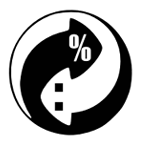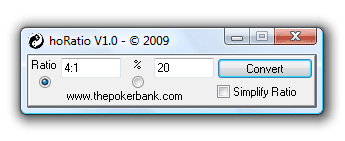# hoRatio - Percentage and Ratio Odds ConverterHere's a nice free poker odds converter program called "hoRatio" for you.## What is hoRatio?

hoRatio is a super quick and lightweight odds converter for poker players. For example, what's 4:1 as a percentage again? Allow hoRatio to help you out.

It converts ratio odds in to percentage odds and percentage odds in to ratio odds, rather than you having to hunt down a percentage to ratio odds converter chart all the time. Just a no-nonsense odds conversion tool for use in poker mathematics.## Why use hoRatio?

Ratio odds and percentage odds are always being interchanged in poker books, strategy articles and poker forums. Strangely enough, neither format has really become the "standard" for working out pot odds, equity and all that mathematical stuff in poker.

As a result, you will often bump in to these two different odds formats in your quest for poker greatness, and it can sometimes be pretty hard to keep up with it all.

So unless you are familiar with odds or it wasn't too long ago that you were in a math class, it's not always easy to convert ratios to percentages and vice versa in your head. So just allow hoRatio to do the leg work for you.

## How to use hoRatio.

Highlight the odds format you want to convert using the radio button in the program, enter the numbers and press "convert".

If you have a tricky ratio for whatever reason like "9 to 4" (basically anything other than x to 1), you can simplify this by ticking the "simplify ratio" box.

### Entering a ratio.

As long as the two numbers in the ratio are separated by a colon ":" or the word "to", hoRatio will be able to convert it in to a percentage for you. Here are a few examples of how you can input a ratio in to the ratio field:

• 5:1
• 7 to 2
• 3to2
• 66: 1

## hoRatio features.

• Converts ratio odds in to percentage odds.
• Converts percentage odds in to ratio odds.
• Simplifies ratio odds also if you like.

## Why is it called hoRatio?

Because it converts ratios in to percentages, and Horatio is a character from William Shakespeare's play Hamlet. Force them together and you get hoRatio.

It also converts percentages in to ratios, but let's not worry about that for now.

If you find hoRatio useful, how about adding some money to Iain's (the developer's) beer fund? A dollar or two here and there is always much appreciated!Cheers!

Go back to the Texas Hold'em software.